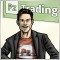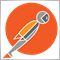# [Same old] Which is the correct way to calculate the lotsize?797

Hello folks,

I'm going around the lotsize calculation and I'm having trouble coming up with a function that works fine for different denominated accounts and 2,3,4 and 5 digit symbols. The following lotsize calculation works, but will it work for accounts denominated in other currencies? I've read WHRoeder posts about lotsize calculations, and I'd like to read his opinion on this.

`double l_lotz = AccountFreeMargin() * RiskPercent/100/((GetStopLossRange(Type)/Point) * MarketInfo(Symbol(), MODE_TICKVALUE));`

Thanks a lot.20069

Account free margin is irrelevant to your risk. Your risk is the (orderOpenPrice - ISL) * DeltaPerPoint * lotsize. Which you want to equal AccountBalance * RiskPercent/100. You solve from there.

You must ALSO compute the free Margin at the most adverse excursion (SL) for ALL open orders (on all charts) including the new one and reduce lotsize further to prevent a margin call.

See my code

Also you can not use tick value alone like that. See my code for DeltaPerPoint().797

Thanks WHR!184

whroeder1:

Account free margin is irrelevant to your risk. Your risk is the (orderOpenPrice - ISL) * DeltaPerPoint * lotsize. Which you want to equal AccountBalance * RiskPercent/100. You solve from there.

You must ALSO compute the free Margin at the most adverse excursion (SL) for ALL open orders (on all charts) including the new one and reduce lotsize further to prevent a margin call.

See my code

Also you can not use tick value alone like that. See my code for DeltaPerPoint().

I can't find coding for a function DeltaPerPoint() in your link. Very interested in this.20069

Risk depends on your initial stop loss, lot size, and the value of the pair.
• You place the stop where it needs to be - where the reason for the trade is no longer valid. E.g. trading a support bounce the stop goes below the support.
• Account Balance * percent/100 = RISK = OrderLots * (|OrderOpenPrice - OrderStopLoss| * DeltaPerLot + CommissionPerLot) (Note OOP-OSL includes the SPREAD, and DeltaPerLot is usually around \$10/pip but it takes account of the exchange rates of the pair vs. your account currency.)
• Do NOT use TickValue by itself - DeltaPerLot and verify that MODE_TICKVALUE is returning a value in your deposit currency, as promised by the documentation, or whether it is returning a value in the instrument's base currency (EUR, in this case).
MODE_TICKVALUE is not reliable on non-fx instruments with many brokers.
• You must normalize lots properly and check against min and max.
• You must also check FreeMargin to avoid stop out
Most pairs are worth about \$10 per PIP. A \$5 risk with a (very small) 5 PIP SL is \$5/\$10/5=0.1 Lots maximum.2391

whroeder1:
Risk depends on your initial stop loss, lot size, and the value of the pair.
• You place the stop where it needs to be - where the reason for the trade is no longer valid. E.g. trading a support bounce the stop goes below the support.
• Account Balance * percent/100 = RISK = OrderLots * (|OrderOpenPrice - OrderStopLoss| * DeltaPerLot + CommissionPerLot) (Note OOP-OSL includes the SPREAD, and DeltaPerLot is usually around \$10/pip but it takes account of the exchange rates of the pair vs. your account currency.)
• Do NOT use TickValue by itself - DeltaPerLot and verify that MODE_TICKVALUE is returning a value in your deposit currency, as promised by the documentation, or whether it is returning a value in the instrument's base currency (EUR, in this case).
MODE_TICKVALUE is not reliable on non-fx instruments with many brokers.
• You must normalize lots properly and check against min and max.
• You must also check FreeMargin to avoid stop out
Most pairs are worth about \$10 per PIP. A \$5 risk with a (very small) 5 PIP SL is \$5/\$10/5=0.1 Lots maximum.

It's not as simple as just checking the free margin... The max-lot calculation to avoid a margin-call is a dynamic function of multiple factors.

```where n = the max number of lots before a margin call at the stoploss (formula not assignment)

(equity - (tick_value * number_of_ticks_to_stop)) * n) / (margin_used + margin_requirement_for_1_lot * n) = margin_call_percect```

Here is a library to calculate lots that is as accurate as possible ... I had to reprogram the tick_value and margin_requirement functions since the ones from MQ are broken. The LotCalc() function returns the minimum of three separate calculations.

1. Standard risk based lot calc
2. Calc max lots to maintain a minimum overall account margin level percentage (default is 200%)
3. Calc max lots to avoid a margin call in the event that the price moves against the position.

(bonus 4066/4073 handling lib included since it is a dependency)

use:

```#include <lotcalc.mqh>

void OnStart()
{
//---
double risk  = 5.0;//%
double lots  = LotCalc(_Symbol, price, stop,risk, min_margin_level_after_trade);
}
```

peek inside:

```double LotCalc(string symbol, double entry_price, double stoploss_price, double risk_percent = 2.0, double min_margin_level = 200.0)
{
risk_percent     /= 100;
min_margin_level /= 100;
// standard risk based lot calc
double sym_min_lot         = SymbolInfoDouble(symbol, SYMBOL_VOLUME_MIN);
double sym_max_lot         = SymbolInfoDouble(symbol, SYMBOL_VOLUME_MAX);
double sym_lot_step        = SymbolInfoDouble(symbol, SYMBOL_VOLUME_STEP);
double sym_tick_val        = TickValue(symbol);
sym_tick_val        = sym_tick_val <= 0.0 ? 1.0 : sym_tick_val;
double acc_balance         = AccountInfoDouble(ACCOUNT_BALANCE);
double amount_to_risk      = acc_balance * risk_percent;
int    num_ticks           = (int)NormalizeDouble(fabs(entry_price - stoploss_price) / sym_tick_size, 0);
double move_val            = num_ticks * sym_tick_val;
if(move_val == 0.0)
return 0.0;
double lots_standard       = amount_to_risk / move_val;
lots_standard       = round(lots_standard / sym_lot_step) * sym_lot_step;
// calc max-lots_standard based on min margin_level after trade
double margin_used         = AccountInfoDouble(ACCOUNT_MARGIN);
double margin_available    = NormalizeDouble((acc_balance / min_margin_level) - margin_used, 2);
double margin_req_per_lot  = MarginRequired(symbol);
double lots_margin_level   = margin_available / margin_req_per_lot;
lots_margin_level   = round(lots_margin_level / sym_lot_step) * sym_lot_step;
// calc max-lots_standard based on stop=loss creating a margin call @ the current account equity
double acc_equity          = AccountInfoDouble(ACCOUNT_EQUITY);
double margin_call         = AccountInfoDouble(ACCOUNT_MARGIN_SO_CALL) / 100.0;
double lots_margin_call    = acc_equity/((margin_call * margin_req_per_lot ) + move_val);
lots_margin_call    = round(lots_margin_call / sym_lot_step) * sym_lot_step;

double res = fmin(fmin(lots_standard, lots_margin_level), lots_margin_call);
if(res < sym_min_lot)
{
Print(__FUNCTION__, " NOT ENOUGH MONEY TO TRADE!");
return 0.0;
}
return res > sym_max_lot ? sym_max_lot : res;
}```
Files:
lotcalc.mqh 8 kb